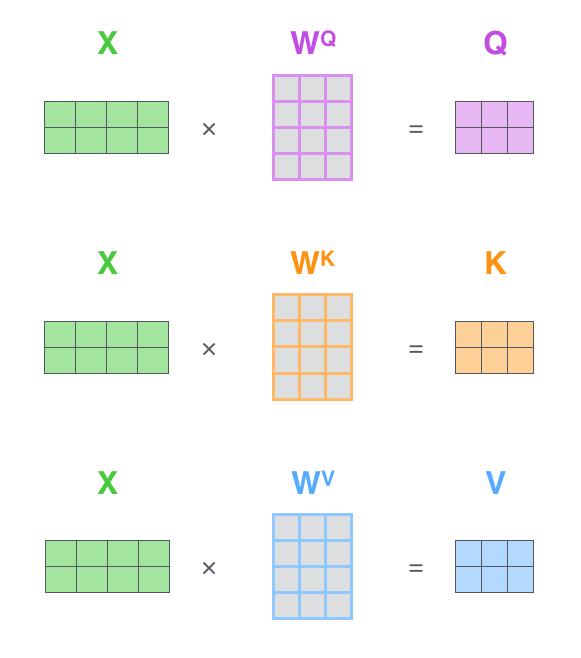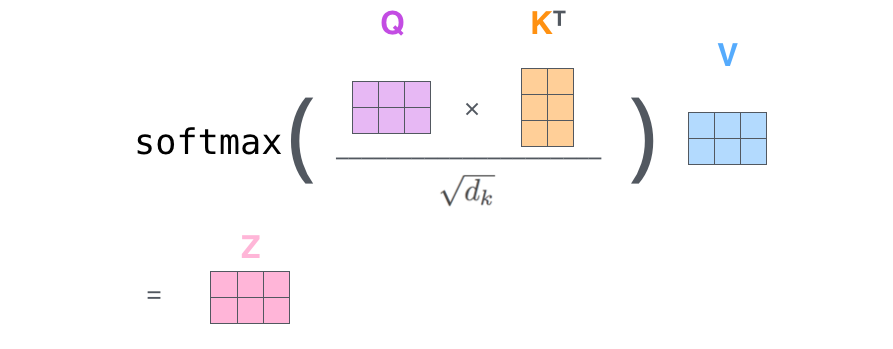# Attention: Isn't it redundant to apply a linear layer to both the keys and values?

Transformer attention is calculated $$Attention(X) =X W^V\times \text{columnwise-softmax} (Att(X))$$ where the attention attention matrix is $$Att(X) = Q \times K = {X W}^Q \times ({X W}^K)^T = {X W}^Q (W^K)^T X^T$$

But then couldn't one of $$W^Q$$ and $$W^K$$ be absorbed into the other? So one of them is redundant say $$W^K$$ and so attention only needs the parameters from $$W^Q$$ and $$W^V$$.

$$Attention(X) = {XW}^V \times \text{columnwise-softmax}({XW}^Q X^T).$$

Diagrams I use for thinking.What I wanted to do wouldn't work because you would be chunking $$X$$. The chunks of $$Q,K,V$$ depend on all the channels of $$X$$, where as the chunks of $$X$$ do not.
q = rearrange(self.w_qs(q), 'b l (head k) -> head b l k', head=self.n_head)